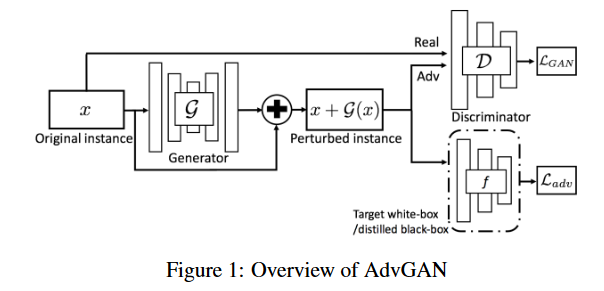# How to backprop the min of loss A and max of loss B?

In the process of training a GAN, I am trying to change the params of gen() such that a cross-entropy loss: `f_loss = CE_loss(f_pred, labels)` is maximized and a different type of loss: `lossG = torch.mean(torch.log(1. - output))` is minimized.

This is my code:

``````adv_ex = adv_ex.reshape(32, 28*28)
lossG = torch.mean(torch.log(1. - output)) #get loss for gen's desired desc pred

f_pred = target(adv_ex) #.size() = [32, 10]
f_loss = CE_loss(f_pred, labels) #add loss for gens desired f pred
print(f_loss)
loss_G_Final = f_loss+lossG # can change the weight of this loss term later

loss_G_Final = loss_G_Final.to(device)
loss_G_Final.backward()
opt_gen.step()
``````

I’m pretty sure that this will minimize lossG, but also minimize f_loss. How can I change this so that the gen() parameters will maximize the crossentropy f_loss as well as minimize lossG?

here is the entire code block if needed:

``````
# set up the optimizers and loss for the models
CE_loss = nn.CrossEntropyLoss()

for epoch in range(num_epochs):
for batch_idx, (real, labels) in enumerate(loader):
#get a fixed input batch to display gen output
if batch_idx == 0:
if epoch == 0:
fixed_input = real.view(-1,784).to(device)

adv_ex = real.clone().reshape(-1,784).to(device) # [32, 784] advex copy of first batch flattened
real = real.view(-1, 784).to(device) # [32, 784] # real batch flattened
labels = labels.to(device) # size()  32 labels in batch

# Train Generator: min log(1 - D(G(z))) <-> max log(D(G(z))
# also max the CE loss between target-model pred and true labels

lossG = torch.mean(torch.log(1. - output)) #get loss for gen's desired desc pred

f_pred = target(adv_ex) #.size() = [32, 10]
f_loss = CE_loss(f_pred, labels) #add loss for gens desired f pred
print(f_loss)
loss_G_Final = f_loss+lossG # can change the weight of this loss term later

loss_G_Final = loss_G_Final.to(device)
loss_G_Final.backward()
opt_gen.step()

# Train Discriminator: max log(D(x)) + log(1 - D(G(z)))

disc_real = disc(real).view(-1)
lossD = -torch.mean(torch.log(disc(real)) + torch.log(1. - disc(adv_ex)))

lossD.backward()
opt_disc.step()
``````

Hi Ryan!

I haven’t looked at you code in detail, and I’m not sure I understand

Let me say what I think you’re trying to do.

First, when a model is doing “better,” its associated loss is lower.
Pytorch’s optimizers, such a `Adam`, seek to minimize the loss.

I assume that `f_loss` is measuring how well your generator, `gen`,
is generating a “fake” sample with certain desired characteristics.
If a lower value of `f_loss` means that `gen` is doing better, then
calling `f_loss.backward()` and `opt_gen.step()` will move `gen`'s
parameters in the direction that improves `gen`'s performance (as
measured by `f_loss`).

Now I assume that `lossG` measures how good your discriminator,
`disc`, is at telling the “fake” samples generated by `gen` from real
samples. Smaller values of `lossG` mean that your discriminator
is working better.

The complexity comes because you want to train `disc` to do well,
that is to lower `lossG`. But you want to train `gen` so that `disc`
has a hard time distinguishing fake from real, that is to increase
`lossG`

Assuming that `disc`'s parameters are (temporarily) frozen, you want
to train `gen` to lower `f_loss` while increasing `lossG`. Doing this is as
simple as training `gen` with (note the minus sign):

``````loss_G_Final = f_loss - lossG
``````

(You may well choose to weight `f_loss` and `lossG` differently to
adjust `gen`'s trade-off in producing the characteristics measured
by `f_loss` vs. tricking `disc`, as measured by `lossG`.)

One final note: When you train `disc` to successfully distinguish
fake from real, you will not want to flip the sign of `lossG`. You
would call:

``````lossG.backward()
opt_disc.step()
``````

`f_loss` doesn’t depend on `disc`'s parameters (unless your code is
hiding something somewhere), so you neither need nor want to call
`f_loss.backward()` (nor `loss_G_Final.backward()`) before calling
`opt_disc.step()`.

Best.

K. Frank

Thanks for the response!

Ideally, F_Loss would be a measure of how well a classifier: F performs on the adversarial examples: x +G(x).
I want the generator: G to generate noise such that F_Loss is HIGH. This would indicate that the noise: G(x) when added to the image: x will cause F to make a misclassification.I also want the generator: G to generate noise: G(x) such that the discriminator: D classifies G(x) + x as a real member of x. ie D(G(x) + x) == 1.

D on the other hand should classify G(x) + x as a fake image ie D(G(x)+x) == 0

The goal of this network is to train G to produce adversarial examples that look like real data, and fool F.

Hi Ryan!

As I understand it, your have three models here: G is `gen` in the
code you posted above, D is `disc`, and F, I suppose, is `target`.

For this part of the training I’ll assume that the parameters of `gen`
and `target` are (temporarily) frozen, and that you are training just
the parameters of `gen`.

You want the model `target` to misclassify, that is (going back to the
code you posted), `f_loss` is large. You also want `disc` to mistake
fake for real, that is, `lossG` is also large.

Pytorch optimizers seek to minimize the losses they’re given, so you
should simply flip the signs of `f_loss` and `lossG` and backpropagate
normally through `gen`. (Based on your clarification that you want `gen`
to fool `target` (as well as `disc`), you should – unlike what I said in my
first reply – also flip the sign of `f_loss`.) Thus:

``````loss_G_Final = - (f_loss + lossG)
procedure, you presumably also want to be training `disc` to do
you train `gen` to keep getting better at tricking `disc`.)